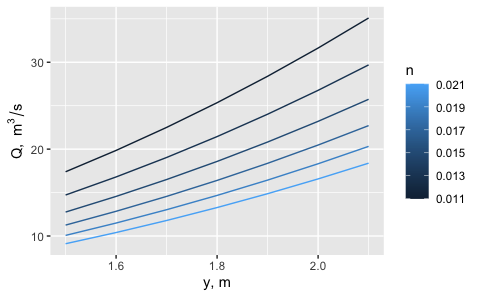# Hydraulics Vignette

#### 2020-08-25

library(hydraulics)

# Introduction

The hydraulics package was developed to augment education in basic closed conduit and open channel hydraulics. Most common applications in civil engineering involve water flowing under turbulent conditions, so the functions make that assumption. If that assumption is violated, a warning is generated, though that often means there was an input error. Because engineering calculations are burdened by the persistence of U.S. customary (often referred to as English) units, all functions work in either system by designating units as either SI or Eng.

## 1.0 Water properties

When describing the behavior of water in pipes and channels under turbulent flow conditions there are three water properties used used in calculations, all of which vary with water temperature: density ($$\rho$$), dynamic viscosity($$\mu$$), and kinematic viscosity($$\nu$$), where they are related by $$\nu=\frac{\mu}{\rho}$$.

These three properties are found using the dens, dvisc, and kvisc functions. For example, the kinematic viscosity for water temperature of 55 F is found as follows:

nu = kvisc(T = 55, units = 'Eng')
cat(sprintf("Kinematic viscosity: %.3e ft2/s\n", nu))
#> Kinematic viscosity: 1.318e-05 ft2/s

Similarly the water density for water temperature of 25 C can be determined by:

rho = dens(T = 25, units = 'SI')
cat(sprintf("Water density: %.3f kg/m3\n", rho))
#> Water density: 997.075 kg/m3

The water property functions can all accept a list of input temperature values, enabling visualization of a property with varying water temperature, for example:

Ts <- seq(0, 100, 10)
nus <- kvisc(T = Ts, units = 'SI')
xlbl <- expression("Temperature, " (degree*C))
ylbl <- expression("Kinematic viscosity," ~nu~ (m^{2}/s))
par(cex=0.8, mgp = c(2,0.7,0))
plot(Ts, nus, xlab = xlbl, ylab = ylbl, type="l")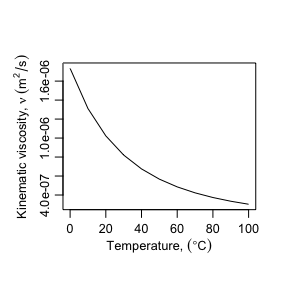The water property functions can also be called with the ret_units parameter, in which case the function returns an object of class units. This enables capabilities for new units being deduced as operations are performed on the values. A concise example of this is in a blog post by Edzer Pebesma, the author of the units package.

T <- 25
Dens <- dens(T = T, units = 'SI', ret_units = TRUE)
Dvisc <- dvisc(T = T, units = 'SI', ret_units = TRUE)
#Calculate kinematic viscosity and units are generated correctly
Kvisc <- Dvisc / Dens
Kvisc
#> 9.134879e-07 [N*m*s/kg]

While the units are correct, they are not in their simplified form. That can be done by setting new units. Unit conversions are done the same way.

units::set_units(Kvisc, m^2/s)
#> 9.134879e-07 [m^2/s]
units::set_units(Kvisc, ft^2/s)
#> 9.832702e-06 [ft^2/s]

If the results are of class units they also can have the units plot without the cumbersome formatting of axis labels.

Temperature <- units::set_units(seq(0, 100, 10), degree_Celsius)
Kinematic_Viscosity <- kvisc(T = Temperature, units = 'SI', ret_units = TRUE)
par(cex=0.8, mar = par("mar") + c(0, .2, 0, 0))
plot(Temperature, Kinematic_Viscosity, type="l")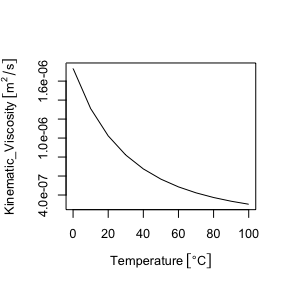## 2.0 Fundamental constants

Two of the most important descriptive quantities in engineering hydraulics are the Reynolds number, Re and the Froude number Fr. $$Re=\frac{VD}{\nu}$$ describes the turbulence of the flow. It expresses the ratio of kinematic forces, expressed by velocity V and a characteristic length such as pipe diameter, D, to viscous forces as expressed by the kinematic viscosity $$\nu$$. For open channels the characteristic length is the hydraulic depth, the area of flow divided by the top width. For adequately turbulent conditions to exists, Reynolds numbers should exceed 4000 for full pipes, and 2000 for open channels.

For open channel flow, given a channel shape and flow rate, flow can usually exist at two different depths, termed subcritical (slow, deep) and supercritical (shallow, fast). The exception is at critical flow conditions, where only one depth exists, the critical depth. Which depth is exhibited by the flow is determined by the slope and roughness of the channel. The Froude number is defined by $$Fr=\frac{V}{\sqrt{gD}}$$. Fr characterizes flow as:

Fr Condition
<1.0 subcritical
=1.0 critical
>1.0 supercritical

These constants are calculated internally and returned with the output of many functions. Critical flow is important in open-channel flow applications and is discussed further below.

## 3.0 Friction Loss in Circular Pipes

The energy at any point along a pipe containing flowing water is often described by the energy per unit weight, or energy head, E: $E = z+\frac{P}{\gamma}+\alpha\frac{V^2}{2g}$ where P is the pressure, $$\gamma=\rho g$$ is the specific weight of water, z is the elevation of the point, V is the average velocity, and each term has units of length. $$\alpha$$ is a kinetic energy adjustment factor to account for non-uniform velocity distribution across the cross-section. $$\alpha$$ is typically assumed to be 1.0 for turbulent flow because the value is close to 1.0 and $$\frac{V^2}{2g}$$ (the velocity head) tends to be small in relation to other terms in the equation.

As water flows through a pipe energy is lost due to friction with the pipe walls and local disturbances (minor losses). The energy loss between two sections is expressed as $${E_1} - {h_l} = {E_2}$$. When pipes are long, with $$\frac{L}{D}>1000$$, friction losses dominate the energy loss on the system, and the head loss, $$h_l$$, is calculated as the head loss due to friction, $$h_f$$. This energy head loss due to friction with the walls of the pipe is described by the Darcy-Weisbach equation, which estimates the energy loss per unit weight, or head loss $${h_f}$$, which has units of length. For circular pipes it is expressed as: $h_f = \frac{fL}{D}\frac{V^2}{2g} = \frac{8fL}{\pi^{2}gD^{5}}Q^{2}$ In this equation f is the friction factor, typically calculated with the Colebrook equation: $\frac{1}{\sqrt{f}} = -2\log\left(\frac{\frac{k_s}{D}}{3.7D} + \frac{2.51}{Re\sqrt{f}}\right)$ where $$k_s$$ is the absolute roughness of the pipe wall. There are close approximations to the Colebrook equation that have an explicit form to facilitate hand-calculations, but this package only uses the Colebrook function.

Any one of the variables in the Darcy Weisbach equation, and by extension the Colebrook equation, may be treated as an unknown. For an existing pipe with a known flow rate, the friction loss for a length of pipe may be found:

D <- 20/12    #20 inch converted to ft
L <- 10560    #ft
Q <- 4        #ft^3/s
T <- 60       #degrees F
ks <- 0.0005  #ft

ans <- darcyweisbach(Q = Q,D = D, L = L, ks = ks,
nu = kvisc(T=T, units="Eng"), units = c("Eng"))
#> hf missing: solving a Type 1 problem
cat(sprintf("Reynolds no: %.0f\nFriction Fact: %.4f\nHead Loss: %.2f ft\n",
ans$Re, ans$f, ans$hf)) #> Reynolds no: 248625 #> Friction Fact: 0.0173 #> Head Loss: 5.72 ft The Reynolds number is adequately high to ensure flow is turbulent and the Colebrook equation is valid. As with the water properties function, it can be called with ret_units = TRUE to return a list of units objects (using the same input as above): ans <- darcyweisbach(Q = 4.0,D = 20/12, L = 10560, ks = 0.0005, nu = kvisc(T=T, units="Eng"), units = "Eng", ret_units = TRUE) knitr::kable(format(as.data.frame(ans), digits = 2), format = "pipe") Q V L D hf f ks Re 4 [ft^3/s] 1.8 [ft/s] 10560 [ft] 1.7 [ft] 5.7 [ft] 0.017  5e-04 [ft] 248625  A new design problem can involve the calculation of a required diameter for a given head loss and flow rate, so that the pipe has a specified pressure and flow at some downstream point. An example of that follows. Q <- 37.5 #flow in ft^3/s L <- 8000 #pipe length in ft hf <- 215 #head loss due to friction, in ft T <- 68 #water temperature, F ks <- 0.0008 #pipe roughness, ft ans <- darcyweisbach(Q = Q, hf = hf, L = L, ks = ks, nu = kvisc(T=T, units='Eng'), units = c('Eng')) #> D missing: solving a Type 3 problem cat(sprintf("Reynolds no: %.0f\nFriction Fact: %.4f\nDiameter: %.2f ft\n", ans$Re, ans$f, ans$D))
#> Reynolds no: 2336974
#> Friction Fact: 0.0164
#> Diameter: 1.85 ft

The usefulness of an R package is not so much for individual calculations, but repeated trials to see how one variable might vary with another. For example, it might be interesting to see how the required diameter varies with changing flow rate. The following example illustrates the calculation of diameters required to meet the specified head loss for flows varying from 30 - 45 ft^3/s.

Qs <- seq(30, 45, 1.0)    #flows in ft^3/s
L <- 8000                 #pipe length in ft
hf <- 215                 #head loss due to friction, in ft
T <- 68                   #water temperature, F
ks <- 0.0008              #pipe roughness, ft
ans <- mapply(darcyweisbach, Q=Qs, MoreArgs =
list(hf = hf, L = L, ks = ks, nu = kvisc(T=T, units='Eng'), units = 'Eng'))
ans <- as.data.frame(t(ans))
plot(ans$Q, ans$D, xlab = "Q, ft^3/s", ylab = "D, ft", type="l")
grid()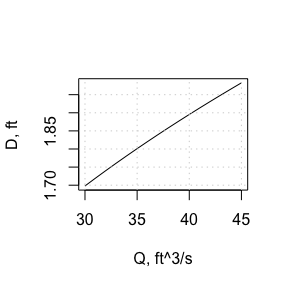Another example of the use of this package would be in a laboratory setting, where pressure measurements are taken at two points along a straight pipe for a sequence of flow rates to determine pipe roughness. In this example, the length of pipe is 3 m, the diameter is 25 mm, and the following head losses were observed for different flow rates:

0.20 0.052
0.24 0.073
0.30 0.110

Roughness values are calculated as shown below, and the results plotted on a Moody diagram as a reality check.

Qs = c(0.00020, 0.00024, 0.00030) #converted to m^3/s
hfs <- c(0.052,0.073,0.110)
ans <- mapply(darcyweisbach, Q=Qs, hf=hfs, MoreArgs =
list(L = 3.0, D = 0.025, nu = kvisc(T=20, units='SI'), units = 'SI'))
ks_values = unlist((as.data.frame(t(ans)))$ks) cat(round(ks_values,6)) #> 0.000473 0.000433 0.000397 cat(paste0("\nMean Roughness, ks = ",round(mean(ks_values),6), " m")) #> #> Mean Roughness, ks = 0.000434 m Re_values <- unlist((as.data.frame(t(ans)))$Re)
f_values <- unlist((as.data.frame(t(ans)))$f) moody(Re = Re_values, f = f_values)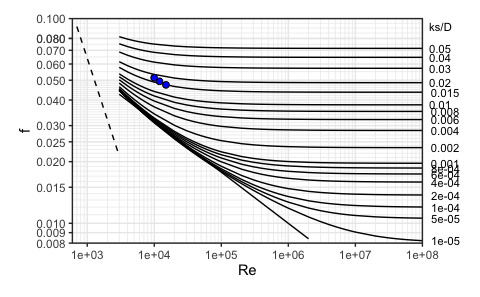## 4.0 Flow in Circular Pipes Flowing Partially Full The Manning equation (also known as the Strickler equation) describes flow conditions in an open channel under uniform flow conditions. It is often expressed as: $Q=A\frac{C}{n}{R}^{\frac{2}{3}}{S_f}^{\frac{1}{2}}$ where C is 1.0 for SI units and 1.49 for Eng (U.S. Customary) units. Q is the flow rate, A is the cross-sectional flow area, n is the Manning roughness coefficient, and R is the hydraulic radius $$R=\frac{A}{P}$$, where P is the wetted perimeter. Critical depth is defined by the relation (at critical conditions): $\frac{Q^{2}B}{g\,A^{3}}=1$ where B is the top width of the water surface. As with full flow in circular pipes, any one of the variables in the Manning equation, and related geometric variables, may be treated as an unknown. For an existing pipe, a common problem is the determination of the depth, y that a given flow Q, will have given a pipe diameter d, slope S and roughness n. An example of that follows. ans <- manningc(Q=0.01, n=0.013, Sf=0.001, d = 0.2, units="SI", ret_units = TRUE) knitr::kable(format(as.data.frame(ans), digits = 2), format = "pipe", padding=0) Q V A P R y d Sf n yc Fr Re Qf 0.01 [m^3/s] 0.38 [m/s] 0.027 [m^2] 0.44 [m] 0.061 [m] 0.16 [m] 0.2 [m] 0.001  0.013  0.085 [m] 0.3  22343  0.01 [m^3/s] It is also sometimes convenient to see a cross-section diagram. ans <- manningc(Q=0.01, n=0.013, Sf=0.001, d = 0.2, units="SI", ret_units = TRUE) xc_circle(y = ans$y, d=ans$d, units = "SI")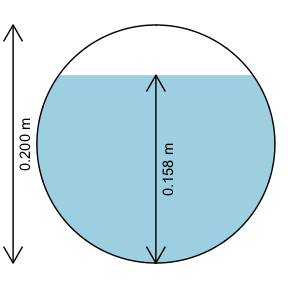## 5.0 Flow in Open Channels (rectangular, triangular, trapezoidal) The manningt function works similarly to the function for circular pipes, in that the excluded argument is the one for which the function determines a solution. For example, a design might require the slope to deliver a required flow, Q through a channel with known geometry (bottom width, b, side slope m) and a given depth y: ans <- manningt(Q = 360., n = 0.015, m = 1, b = 20.0, y = 3.0, units = "Eng") cat(sprintf("Slope: %.5f ft\n", ans$Sf))
#> Slope: 0.00088 ft
knitr::kable(format(as.data.frame(ans), digits = 2), format = "pipe", padding=0)
Q V A P R y b m Sf B n yc Fr Re
360 5.2 69 28 2.4 3 20 1 0.00088 26 0.015 2.1 0.56 3.7e+07

Thus, a longitudinal slope for the channel would need to be 0.00088, or a drop of 0.88 ft per 1000 ft. The critical depth yc is lower than the normal depth y, indicating flow under these conditions is subcritical, also seen with Fr < 1.0.

Units can also be returned by this function.

ans <- manningt(Q = 360., n = 0.015, m = 1, b = 20.0, y = 3.0, units = "Eng", ret_units = TRUE)
knitr::kable(format(as.data.frame(ans), digits = 2), format = "pipe", padding=0)
Q V A P R y b m Sf B n yc Fr Re
360 [ft^3/s] 5.2 [ft/s] 69 [ft^2] 28 [ft] 2.4 [ft] 3 [ft] 20 [ft] 1  0.00088  26 [ft] 0.015  2.1 [ft] 0.56  3.7e+07 

A simple diagram can be generated for the channel.

xc_trap( y = 3.0, b = 20.0, m = 1.0, units = "Eng")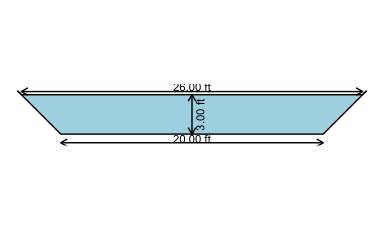For any channel geometry and flow rate a convenient plot is a specific energy diagram, which illustrates the different flow depths that can occur for any given specific energy. This is important for understanding what may happen to the water surface when flow encounters an obstacle or transition. For the channel of the example above, the diagram is

spec_energy_trap( Q = 360, b = 20, m = 1, scale = 4, units = "Eng" )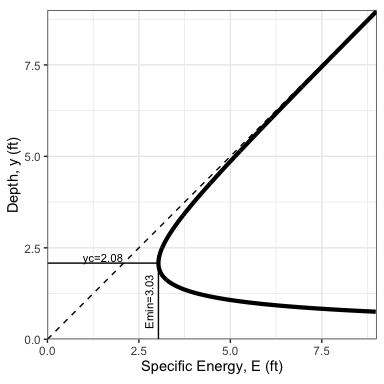This provides an illustration that for y=3 ft the flow is subcritical (above the critical depth). Specific energy for the conditions of the prior example is $$E=y+\frac{V^2}{2g}=3.0+\frac{5.22^2}{2*32.2}=3.42 ft$$. If the channel bottom had an abrupt rise of $$E-E_c=3.42-3.03=0.39 ft$$ critical depth would occur over the hump. A rise of anything greater than that would cause damming to occur. Once flow over a hump is critical, downstream of the hump the flow will be in supercritical conditions, flowing at the alternate depth.

The specific energy for a given depth y and alternate depth can be added to the plot by including an argument for depth, y.

spec_energy_trap( Q = 360, b = 20, m = 1, scale = 4, y=3.0, units = "Eng" )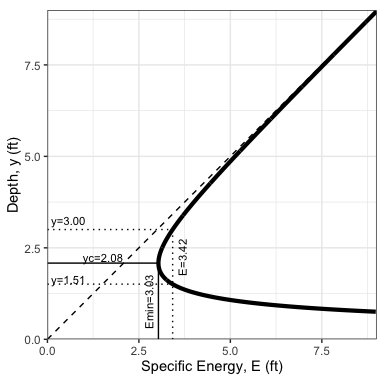A final example shows how to vary multiple input variables simultaneously. How does flow Q vary over a range of n and y values? The expand.grid function produces all combinations of different variables. The functions can be run for all of the problem permutations and the results plotted in many different ways. One example follows.

ns <- seq(0.011, 0.021, 0.002)
ys <- seq(1.5, 2.1, 0.1)
ny <- expand.grid(n=ns, y=ys)
ans <- mapply(manningt, n = ny$n, y = ny$y, MoreArgs = list(m = 2, Sf = 0.0005, b = 3, units = "SI"))
x <- as.data.frame(t(ans))
#to simplify plotting, select columns of interest and change each from list to numeric
x2 <- data.frame(Q=unlist(x$Q),y=unlist(x$y),n=unlist(x\$n))
ggplot2::ggplot(data=x2,ggplot2::aes(x=y,y=Q, group=n, colour=n)) + ggplot2::geom_line() +
ggplot2::labs(x = "y, m", y = expression(paste("Q, ", ~m^3/s)))